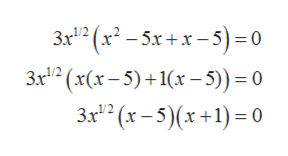Solve the equation by factoring. (Hint: First factor out a fractional power. Enter your answers as a comma-separated list.)3x5/2− 12x3/2= 15x1/2

Question

Solve the equation by factoring. (Hint: First factor out a fractional power. Enter your answers as a comma-separated list.)

3x5/2− 12x3/2= 15x1/2

Step 1

Moving all the terms to one side of the equation.

Step 2

Taking the common term (3x1/2) out from above equation.

Step 3

In the bracket splitting the middle term into two parts such that their product is equal to product...help_outlineImage TranscriptioncloseЗr"" (x - 5x+*-5) -0 Зx"? (х(х - 5) + 1(х - 5)) %3D0 3x" (х -5) (х+1) -0 fullscreen

Want to see the full answer?

See Solution

Want to see this answer and more?

Our solutions are written by experts, many with advanced degrees, and available 24/7

See Solution
Tagged in

Functions